# Abstracts

In this paper we give some examples of surfaces in H³ with conformal normal Gauss map with respect to the second conformal structure and prove some global properties.

hyperbolic space; conformal normal Gauss map; ruled surface; translational surface

Neste trabalho, damos alguns exemplos de superfícies no espaço hiperbólico de dimensão três com aplicação de Gauss conforme relativamente à segunda estrutura conforme e provamos algumas propriedades globais.

espaço hiperbólico; aplicação normal de Gauss conforme; superfície regrada; superfície de translação

MATHEMATICAL SCIENCES

New examples of surfaces in H3 with conformal normal Gauss map

Shuguo Shi

School of Mathematics and System Sciences, Shandong University, Jinan 250100, P.R. China

ABSTRACT

In this paper we give some examples of surfaces in H3 with conformal normal Gauss map with respect to the second conformal structure and prove some global properties.

Key words: hyperbolic space, conformal normal Gauss map, ruled surface, translational surface.

RESUMO

Neste trabalho, damos alguns exemplos de superfícies no espaço hiperbólico de dimensão três com aplicação de Gauss conforme relativamente à segunda estrutura conforme e provamos algumas propriedades globais.

Palavras-chave: espaço hiperbólico, aplicação normal de Gauss conforme, superfície regrada, superfície de translação.

It is well known that the Weierstrass representation formula has played an important role in studying minimal surfaces in R3. To find a Weierstrass-type representation formula for simply connected immersed minimal surfaces in hyperbolic n-space, Kokubu (Kokubu 1997) considered the hyperbolic space as a Lie group G with a left invariant metric. Given a two-dimensional domain D and a map (j) into the above Lie group, he considered the pullback (ji) of the Maurer-Cartan forms to D. Using the standard harmonic map equation for such maps, and by pulling back the Maurer-Cartan structure equations, he derived a complete integrability condition for the 1-forms (ji). Also, to assure that the associated harmonic map is a minimal surface, a conformality condition is imposed. The normal Gauss map into the hyperquadric is written down, which is the usual tangential Gauss map translated to the Lie algebra of G. On the other hand, Gálvez and Martínez (Gálvez and Martínez 2000) studied the properties of the Gauss map of a surface S immersed into the Euclidean 3-space R3, particularly those related to the geometry of the immersion and the so-called second conformal structure of the surface, that is, the conformal structure on S induced by the second fundamental form. Motivated by their work, the author (Shi 2004) gave a Weierstrass representation formula for surfaces with prescribed normal Gauss map and Gauss curvature in H3 by using the second conformal structure on surfaces (see section 1 for the definition). From this, surfaces whose normal Gauss map are conformal maps have been found(see Theorem 1).

The propose of this paper is to classify locally the ruled surfaces with conformal normal Gauss map in H3 and also give some new examples of complete properly embedded ruled surfaces. The rest of the paper is divided into three sections. The first one describes the definitions of the normal Gauss map in context of this paper and the second conformal structure on surfaces and states Theorem 1. The second section gets two local examples within the Euclidean ruled surface and in the last section some global properties of the ruled surfaces and translational surfaces are proven.

1 PRELIMINARIES

Take the upper half-space model of the hyperbolic 3-space H3 = {(x1, x2, x3) Î R3 : x3 > 0} with the Riemannian metric ds2 =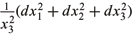and constant sectional curvature -1.

Let S be a connected 2-dimensional smooth surface and x : S ® H3 be an immersion of S into H3 with local coordinates u, v. The first and the second fundamental forms of the immersion are written, respectively, as I = Edu2 + 2Fdudv + Gdv2 and II = Ldu2 + 2Mdudv + Ndv2. The unit normal vector field of x(S) in H3 is written as

where= 1. The Gauss equation is

Identifying H3 with the Lie group

the multiplication is defined as matrix multiplication and the unit element is e = (0,0,1). The Riemannian metric is left-invariant andare the left-invariant unit orthonormal vector fields. Now, the unit normal vector field of x(S) can be written as= e31X1+e32X2+e33X3. Left translatingto Te(H3), we obtain

By the stereographic projection, we get the map S ® C{¥},

Call g1(or g2) the normal Gauss map of surface x(S) (Kokubu 1997). On U1

U2, g1g2 = 1. In this paper, we only consider g1 and write the normal Gauss map as g : S ® C{¥}. Then we have

Consider an immersion x : S ® H3 with Gauss curvature K > -1. By the Gauss equation, we can choose a suitable orientation on x(S) such that the second fundamental form II becomes a positive definite metric on S and induces a conformal structure on S, which is called the second conformal structure like (Klotz 1963). S will be considered as a Riemannian surface with the second conformal structure.

THEOREM 1 (Shi 2004). Let S be a connected Riemannian surface and x : S ® H3 be an immersion with Gauss curvature K > -1. Assume that the set of umbilics has no interior point. Then normal Gauss map g : S ® C

{¥} of x(S) is conformal map if and only if the Gauss curvature K and the normal Gauss map g satisfy.

The Weierstrass representation formulas for these surfaces can be found in (Shi 2004).

REMARK. For the Bryant's hyperbolic Gauss map of surface in H3(see (Bryant 1987) for the definition), Gálvez, Martínez and Milán (Gálvez et al. 2000) proven that the hyperbolic Gauss map is conformal if and only if the surface is either flat or totally umbilic.

Locally, the graph (u, v, f(u, v)) satisfyingmust satisfy the following fully nonlinear PDE of second order (Shi 2004),2 EXAMPLES

We consider the surfaces in H3 as those ones in R3. The simplest examples of surfaces with conformal normal Gauss map are the equidistant surfaces and horospheres x3 = const > 0, i.e. ordinary Euclidean planes. They are totally umbilics with constant Gauss curvature and constant normal Gauss map.

The following theorem gives all the ruled surfaces with conformal normal Gauss map in H3.

THEOREM 2. Up to an isometric transformation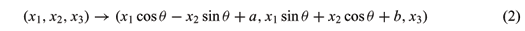of H 3 , every ruled surface with conformal normal Gauss map in H 3 is locally a part of one of the following,

(1) equidistant surface with respect to a vertical hyperbolic plane,

(2) Horosphere x3 = constant > 0,

(3) (u cos v, c · sin v, u sin v), for a constant c ¹ 0,

(4) (-c2 sin v + ucos v, c1 · sin v, c2 cos v + u sin v), for constants c1¹ 0 and c2¹ 0.

REMARK. We may check that, among all isometric transformations of H3 (Korevaar et al. 1992), the horizontal Euclidean translations and rotations (2), the hyperbolic reflections with respect to a vertical hyperplane and the vertical hyperbolic translations

(x1, x2, x3) ® (l(x1 - a), l(x2 - b), lx3), (l > 0),

preserve the concept of the ruled surfaces and the conformality of the normal Gauss map of any surface with conformal normal Gauss map and the hyperbolic reflections

preserve the conformality of the normal Gauss map of all totally umbilics but totally geodesics.

PROOF. Generally, considered as surfaces in R3, the ruled surfaces in H3 can be represented as

x(u, v) = a(v) + ub(v) : D ® H3,

where DR2) is a parameter domain and a(v) and b(v) are two vector value functions into R3 corresponding to two curves in R3.

First, we assume that b is locally nonconstant and without loss of generality assume that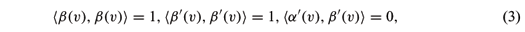where á·,·ñ is the inner product in R3. Write as e = (0,0,1). We have x3 = áx, eñ and b¢¢ ¹ 0. The unit normal vector of the surface x(u, v) : D ® H3 is given by

where X Ù Y is the exterior product of the vectors X and Y in R3 and |·| is the Euclidean norm in R3. So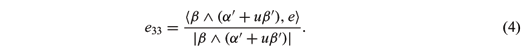By a straight computation, we have,

By the Gauss equation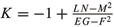and (4), we know thatis equivalent to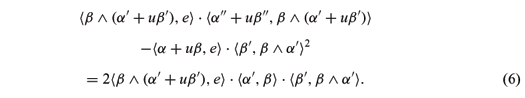Expanding the above formula and noting the linearly independence of 1, u, u2 and u3, we getBy (7), if áb Ù b¢, e ñ ¹ 0 at a point p0, then áb¢¢, b Ù b¢ñ = 0 in a neighbourhood U of p0 and the curver b(v) is a geodesic of S2. Hence, áb Ù b¢, eñ is locally constant and so is globally constant.

CASE 1. If áb Ù b¢, eñ = 0, then áb Ù b¢¢, eñ = 0. Hence b, b¢, b¢¢ and e lie on same plane. We get áb, b¢Ù b¢¢ñ = 0. So the curve b(v) is a plane curve in R3, i.e. a unit circle, and the plane p on which b lies passes through the origin of R3. By áb Ù b¢, eñ = 0, we know p is a vertical plane on which x3-axis lies. Revolving p around x3-axis, i.e. making an isometric transformation (x1, x2, x3) ® (x1 cos q - x2 sin q, x1 sin q + x2 cos q, x3), we may assume that p is the plane x1ox3. So b(v) = (cos v, 0, sin v). Assume a(v) = ( x1(v), x2(v), x3(v)), then equation systems (3) and (7)-(10) may be written as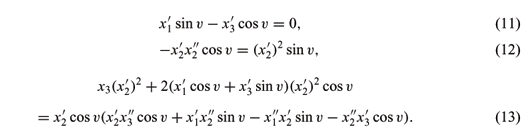Solving (11)(12)(13), we get

a(v) = (-c2 sin v + c3, c1 sin v + c4, c2 cos v),

where c1¹ 0, c2,c3 and c4 are constants. Making a translation (x1, x2, x3) ® (x1 + c3, x2+c4, x3), we get (3) and (4) of Theorem 2.

CASE 2. If áb Ù b¢, eñ ¹ 0, then áb¢¢, b Ù b¢ñ º 0. We also get that the curve b is a plane curve in R3 and the plane p on which the curve b lies passes through the origin of R3 but is not totally geodesic. Similar to case 1, making a rotation transformation around x3-axis, we may assume that x1-axis lies on p. Now, b(v)is a unit circle on p. b(v) = (cos v, sin v cos q, sin v sin q), where constant q is the angle between plane x1ox2 and p. By (8), we get áa¢¢, b Ù b¢ñ = 0. Multiplying (10) by áb Ù b¢, eñ and using (9), we get

áa, eñ · áb Ù b¢, e ñ · áb¢ , b Ù a¢ñ2 = áb Ù a¢, eñ · áb, eñ · áb¢, b Ù a¢ñ2.

Then, according to whether áb¢, b Ù a¢ñ = 0 or not, we again get two cases from equation systems (3) and (7)-(10),

CASE (i),

áa¢, b¢ñ = 0, áb Ù b¢, eñ ¹ 0, áa¢¢, b Ù b¢ñ = 0,

áb¢, b Ù a¢ñ = 0, áa¢¢, b Ù a¢ñ = 0,

and

CASE (ii),

áa¢, b¢ñ = 0, áb Ù b¢, eñ ¹ 0, áa¢¢, b Ù b¢ñ = 0, áb¢, b Ù a¢ñ ¹ 0,

áa, eñ · áb Ù b¢, eñ = áb, eñ · áb Ù a¢, eñ,

áb Ù b¢, eñ · áa¢¢, b Ù a¢ñ - 2áa¢, bñ · áb¢, b Ù a¢ñ · áb Ù b¢, eñ

= áb, eñ · áb¢, b Ù a¢ñ2.

In the case (i), taking derivative on áa¢¢, b Ù b¢ñ = 0, we get áa¢¢¢, b Ù b¢ñ = 0. So áa¢ Ù a¢¢, a¢¢¢ ñ = 0, i.e. the torsion of the curve a(v) is zero. We infer that the curve a(v) is a plane curve and the plane on which the curve a lies parallels to p. Hence x satisfies either (1) or (2) of Theorem 2. As for the case (ii), similar to the proof in the case 1, we know that this is a contradictory system of equations.

Next, when b is constant, we have b Ù e ¹ 0. Or else, by proposition 34 in chapter 7 of (Spivak 1979), we have K º -1. A contradiction. We may assume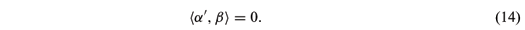Thus a(v) is a plane curve and (6) becomes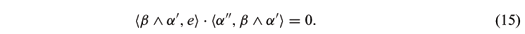We have áb Ù a¢, eñ ¹ 0. Or else, a(v) is a plane curve and lies on a plane parallel to the one spanned by b and e. Then x : D ® H3 is totally geodesic plane H2 and K º -1. This is contradictory to K > -1. So áa¢¢, b Ù a¢ñ = 0. By (14) , we know that a(v) is a straight line in R3 and x : D ® H3 is either a equidistant surface or a horosphere x3 = constant > 0. We have proved Theorem 2.

Locally, the ruled surfaces (3) and (4) in Theorem 2 can be represented as a graph(u, v ,f(u, v)).

COROLLARY 1. f(u, v) =is a solution of equation (1), where c1 ¹ 0 and c2 are constants.

3 GLOBAL PROPERTIES

The equidistant surfaces and horosphere x3 = const > 0 are simply connected, complete, totally umbilics and properly embedded surfaces. Generally, we have the following global properties for the ruled surfaces obtained in theorem 2.

THEOREM 3. The following are simply connected, complete and properly embedded surfaces in H3,

(1) x(u, v) = (u cos v, c · sin v, u sin v) : D1 = {(u, v)|0 < u < +¥, 0 < v < p} ® H3, and

(2) x(u, v) = ( -c2 sin v + u cos v, c1 sin v, c2 cos v + u sin v):

where c > 0, c1 > 0 and c2 > 0 are constants.

PROOF.

(1) The map x : D1® H3 is one-to-one. For any compact subset S in H3, it is easy to know that the relative closed set x-1(S)( Ì Ì D1), as the subset of R2, is bounded and closed set of R2. Hence x-1(S) is a compact set. So, x : D1 ® H3 is a proper map and x(D1) is a complete surface in H3. On the basis of this, we can infer that x : D1® r(D1) is closed. So x maps D1 homeomorphically onto its image with the induced topology and x : D1 ® H3 is embedded.

(2) By a parameter transformation u =and v =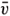+ arctan-, D2 is diffeomorphic to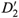= {(,)|-¥ << +¥, 0 << p}. Then x : D2 ® H3 becomes x :® H3 with

Similar to the proof of (1), we can prove (2).

We have obtained the translational surfaces with conformal normal Gauss map in nonparameter form (Shi 2004),

satisfying (1). Now, the parameter form of these translational surfaces is locally given by x(u, v) = (a cos u, b cos v, a sin u + b sin v).

THEOREM 4.

(1) The map x(u,v) = (cos u, cos v, sin u + sin v) : D1® H3 is a simply connected, complete and properly embedded surface, where D1 is a simply connected open domain of R2 enclosed by four straight lines v = u ± p, v = -u + 2p and v = -u.

(2) For 0 < a < b, the image of the map x(u,v) = (a cos u, b cos v, a sin u + b sin v) : D2 ® H3 is a complete and properly embedded surface diffeomorphic to S1 × R, where

PROOF.

(1) By a parameter transformation u =+and v =-, D1 is diffeomorphic to= {(,)|0 << p,-<<} Ì R2. Then x : D1 ® H3 becomes x :® H3 with

Similar to the proof of (1) in Theorem 3, we can prove (1).

(2) It is easy to know that image x(D2) is diffeomorphic to S1 × R . For any divergent curve a : [0, 1) ® D2, when t ® 1-, a(t) tends to either the boundary curve, v = -arcsin (sin u) and v = p + arcsin (sin u) or ¥. For the former, if the length of x(a(t)) is finite, then there exist a compact set S in H3 containing completely the curve x(a(t)). However, when restricting x(D2) on S, there exists a positive constant e0 such that a sin u + b sin v > e0. We may assume e0 < min {a,b-a}. So a(t) must satisfy -arcsin (sin u -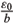) < v < p + arcsin (sin u -). So a(t) does not tend to the boundary of D2, which is contradictory that a(t) tends to the boundary of D2. So the length of x(a(t)) is infinite. For the latter, as t ® 1-, u(t) ® ¥. The first fundamental form of x : D2® H3 satisfies

So the length of x(a(t))

Noting that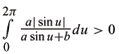, we have L = +¥. In a word, the length of x(a(t)) is always infinite. Hence the image x(D2) is complete.

Next, we prove that the image x(D2) is a proper surface. When restricting x(D2) on any compact set S in H3 mentioned above, the first fundamental form of x : D2 ® H3 satisfies ds2<(du2 + dv2), where C is constant and depend only on a and b. So, we infer that the restriction of S to x(D2) is bounded closed subset of x(D2) and hence is compact subset of x(D2). We complete the proof.

REMARK. The curves in Theorem 3, corresponding respectively to v =on x(D1) and to v = ±on x(D2) and the curves in Theorem 4, corresponding to u = 0,. u = p, v = 0 and v = p on x(D1) and x(D2) are all geodesics of H3 follow which K = -1 and accordingly the second fundamental forms are degenerate. Furthermore, every such a geodesic is mapped to a point by the normal Gauss map.

ACKNOWLEDGMENTS

The author is very grateful to Professor Detang Zhou for his unending concern, support and valuable help. The author would like to express his appreciations to the referees for their interest, careful examination and valuable suggestions.

This work is supported by the starting foundation of science research of Shandong University.

Manuscript received on June 17, 2005; accepted for publication on October 26, 2005; presented by MANFREDO DO CARMO

E-mail: shishuguo@hotmail.com

AMS Classification(2000): 53C42, 53A10

• BRYANT RL. 1987. Surfaces of mean curvature one in hyperbolic space. Astérisque 154-155: 321-347.
• GÁLVEZ JA AND MARTÍNEZ A. 2000. The Gauss map and second fundamental form of surfaces in R3 Geometriae Dedicata 81: 181-192.
• GÁLVEZ JA, MARTÍNEZ A AND MILÁN F. 2000. Flat surfaces in the hyperbolic 3-space. Math Ann 316: 419-435.
• KLOTZ T. 1963. Some uses of the second conformal structure on strictly convex surfaces. Proc Amer Math Soc 14: 793-799.
• KOKUBU M. 1997. Weierstrass representation for minimal surfaces in hyperbolic space. Tôhoku Math J 49: 367-377.
• KOREVAAR NJ, KUSNER R, MEEKS III WH AND SOLOMON B. 1992. Constant mean curvature surfaces in hyperbolic space. Amer J Math 114: 1-43.
• SHI SG. 2004. Weierstrass representation for surfaces with prescribed normal Gauss map and Gauss curvature in H3 Chin Ann Math B 25: 567-586.
• SPIVAK M. 1979. A comprehensive introduction to differential geometry. Publish or Perish Inc, Vol. 4.

# Publication Dates

• Publication in this collection
08 Mar 2006
• Date of issue
Mar 2006

• Accepted
26 Oct 2005